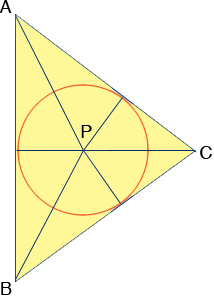SEARCH HOMEMath Central Quandaries & QueriesQuestion from Elle, a student: In the figure, P is the incenter of triangle ABC, the radius of the inscribed circle is 6cm, and the perimeter of triangle ABC is 38cm. What is the area of triangle ABC?Hi Elle,The triangle ABC can be cut into three triangles, ABP, BCP and CAP. Write an expression for the area of each of these triangles. Add them to obtain an expression for the area of triangle ABC. Simplify the expression.

PennyMath Central is supported by the University of Regina and The Pacific Institute for the Mathematical Sciences.